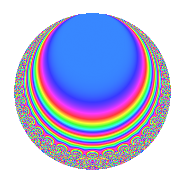Properties

 Label 128.5.d.dLevel 128 Weight 5 Character orbit 128.d Analytic conductor 13.231 Analytic rank 0 Dimension 8 CM No Inner twists 4

Related objects

Newspace parameters

 Level: $$N$$ = $$128 = 2^{7}$$ Weight: $$k$$ = $$5$$ Character orbit: $$[\chi]$$ = 128.d (of order $$2$$ and degree $$1$$)

Newform invariants

 Self dual: No Analytic conductor: $$13.2313552747$$ Analytic rank: $$0$$ Dimension: $$8$$ Coefficient field: 8.0.1871773696.1 Coefficient ring: $$\Z[a_1, \ldots, a_{7}]$$ Coefficient ring index: $$2^{38}$$ Sato-Tate group: $\mathrm{SU}(2)[C_{2}]$

$q$-expansion

Coefficients of the $$q$$-expansion are expressed in terms of a basis $$1,\beta_1,\ldots,\beta_{7}$$ for the coefficient ring described below. We also show the integral $$q$$-expansion of the trace form.

 $$f(q)$$ $$=$$ $$q$$ $$-\beta_{1} q^{3}$$ $$-\beta_{2} q^{5}$$ $$-\beta_{3} q^{7}$$ $$+ ( 55 - \beta_{6} ) q^{9}$$ $$+O(q^{10})$$ $$q$$ $$-\beta_{1} q^{3}$$ $$-\beta_{2} q^{5}$$ $$-\beta_{3} q^{7}$$ $$+ ( 55 - \beta_{6} ) q^{9}$$ $$+ ( -\beta_{1} + \beta_{5} ) q^{11}$$ $$+ ( -4 \beta_{2} - \beta_{4} ) q^{13}$$ $$+ ( 7 \beta_{3} + \beta_{7} ) q^{15}$$ $$+ ( -42 + \beta_{6} ) q^{17}$$ $$+ ( -9 \beta_{1} + 5 \beta_{5} ) q^{19}$$ $$+ ( 13 \beta_{2} + 5 \beta_{4} ) q^{21}$$ $$+ ( -15 \beta_{3} + \beta_{7} ) q^{23}$$ $$+ ( -351 + 6 \beta_{6} ) q^{25}$$ $$+ ( -74 \beta_{1} + 9 \beta_{5} ) q^{27}$$ $$+ ( 17 \beta_{2} - 12 \beta_{4} ) q^{29}$$ $$+ 3 \beta_{7} q^{31}$$ $$+ ( 104 - 5 \beta_{6} ) q^{33}$$ $$+ ( -88 \beta_{1} + 10 \beta_{5} ) q^{35}$$ $$+ ( 4 \beta_{2} + 25 \beta_{4} ) q^{37}$$ $$+ ( 37 \beta_{3} + 4 \beta_{7} ) q^{39}$$ $$+ ( 498 - 6 \beta_{6} ) q^{41}$$ $$+ ( 159 \beta_{1} + 10 \beta_{5} ) q^{43}$$ $$+ ( -146 \beta_{2} - 43 \beta_{4} ) q^{45}$$ $$+ ( -42 \beta_{3} - 3 \beta_{7} ) q^{47}$$ $$+ 737 q^{49}$$ $$+ ( 142 \beta_{1} - 9 \beta_{5} ) q^{51}$$ $$+ ( 66 \beta_{2} + 51 \beta_{4} ) q^{53}$$ $$+ 43 \beta_{3} q^{55}$$ $$+ ( 1064 - 29 \beta_{6} ) q^{57}$$ $$+ ( -393 \beta_{1} - 36 \beta_{5} ) q^{59}$$ $$+ ( 68 \beta_{2} - 61 \beta_{4} ) q^{61}$$ $$+ ( -55 \beta_{3} - 13 \beta_{7} ) q^{63}$$ $$+ ( -3696 + 34 \beta_{6} ) q^{65}$$ $$+ ( -33 \beta_{1} - 31 \beta_{5} ) q^{67}$$ $$+ ( 59 \beta_{2} + 67 \beta_{4} ) q^{69}$$ $$+ ( -69 \beta_{3} - 17 \beta_{7} ) q^{71}$$ $$+ ( -2458 + 63 \beta_{6} ) q^{73}$$ $$+ ( 951 \beta_{1} - 54 \beta_{5} ) q^{75}$$ $$+ ( 65 \beta_{2} - 39 \beta_{4} ) q^{77}$$ $$+ 134 \beta_{3} q^{79}$$ $$+ ( 5321 - 29 \beta_{6} ) q^{81}$$ $$+ ( 567 \beta_{1} - 60 \beta_{5} ) q^{83}$$ $$+ ( 133 \beta_{2} + 43 \beta_{4} ) q^{85}$$ $$+ ( -11 \beta_{3} - 17 \beta_{7} ) q^{87}$$ $$+ ( -3354 + 23 \beta_{6} ) q^{89}$$ $$+ ( -456 \beta_{1} + 14 \beta_{5} ) q^{91}$$ $$+ ( -408 \beta_{2} - 24 \beta_{4} ) q^{93}$$ $$+ ( 243 \beta_{3} + 4 \beta_{7} ) q^{95}$$ $$+ ( -2730 - 87 \beta_{6} ) q^{97}$$ $$+ ( -523 \beta_{1} - 36 \beta_{5} ) q^{99}$$ $$+O(q^{100})$$ $$\operatorname{Tr}(f)(q)$$ $$=$$ $$8q$$ $$\mathstrut +\mathstrut 440q^{9}$$ $$\mathstrut +\mathstrut O(q^{10})$$ $$8q$$ $$\mathstrut +\mathstrut 440q^{9}$$ $$\mathstrut -\mathstrut 336q^{17}$$ $$\mathstrut -\mathstrut 2808q^{25}$$ $$\mathstrut +\mathstrut 832q^{33}$$ $$\mathstrut +\mathstrut 3984q^{41}$$ $$\mathstrut +\mathstrut 5896q^{49}$$ $$\mathstrut +\mathstrut 8512q^{57}$$ $$\mathstrut -\mathstrut 29568q^{65}$$ $$\mathstrut -\mathstrut 19664q^{73}$$ $$\mathstrut +\mathstrut 42568q^{81}$$ $$\mathstrut -\mathstrut 26832q^{89}$$ $$\mathstrut -\mathstrut 21840q^{97}$$ $$\mathstrut +\mathstrut O(q^{100})$$

Basis of coefficient ring in terms of a root $$\nu$$ of $$x^{8}\mathstrut +\mathstrut$$ $$31$$ $$x^{4}\mathstrut +\mathstrut$$ $$81$$:

 $$\beta_{0}$$ $$=$$ $$1$$ $$\beta_{1}$$ $$=$$ $$($$$$-4 \nu^{7} - 18 \nu^{5} - 286 \nu^{3} + 414 \nu$$$$)/189$$ $$\beta_{2}$$ $$=$$ $$($$$$-44 \nu^{6} - 752 \nu^{2}$$$$)/63$$ $$\beta_{3}$$ $$=$$ $$($$$$80 \nu^{7} + 72 \nu^{5} + 2696 \nu^{3} + 4392 \nu$$$$)/189$$ $$\beta_{4}$$ $$=$$ $$($$$$12 \nu^{6} + 368 \nu^{2}$$$$)/7$$ $$\beta_{5}$$ $$=$$ $$($$$$-272 \nu^{7} + 504 \nu^{5} - 7352 \nu^{3} + 12600 \nu$$$$)/189$$ $$\beta_{6}$$ $$=$$ $$($$$$64 \nu^{4} + 992$$$$)/7$$ $$\beta_{7}$$ $$=$$ $$($$$$-1024 \nu^{7} - 2304 \nu^{5} - 24832 \nu^{3} - 43776 \nu$$$$)/189$$
 $$1$$ $$=$$ $$\beta_0$$ $$\nu$$ $$=$$ $$($$$$\beta_{7}\mathstrut +\mathstrut$$ $$4$$ $$\beta_{5}\mathstrut +\mathstrut$$ $$32$$ $$\beta_{3}\mathstrut +\mathstrut$$ $$112$$ $$\beta_{1}$$$$)/1024$$ $$\nu^{2}$$ $$=$$ $$($$$$11$$ $$\beta_{4}\mathstrut +\mathstrut$$ $$27$$ $$\beta_{2}$$$$)/256$$ $$\nu^{3}$$ $$=$$ $$($$$$5$$ $$\beta_{7}\mathstrut +\mathstrut$$ $$4$$ $$\beta_{5}\mathstrut +\mathstrut$$ $$64$$ $$\beta_{3}\mathstrut -\mathstrut$$ $$272$$ $$\beta_{1}$$$$)/512$$ $$\nu^{4}$$ $$=$$ $$($$$$7$$ $$\beta_{6}\mathstrut -\mathstrut$$ $$992$$$$)/64$$ $$\nu^{5}$$ $$=$$ $$($$$$-$$$$61$$ $$\beta_{7}\mathstrut +\mathstrut$$ $$92$$ $$\beta_{5}\mathstrut -\mathstrut$$ $$608$$ $$\beta_{3}\mathstrut -\mathstrut$$ $$2800$$ $$\beta_{1}$$$$)/1024$$ $$\nu^{6}$$ $$=$$ $$($$$$-$$$$47$$ $$\beta_{4}\mathstrut -\mathstrut$$ $$207$$ $$\beta_{2}$$$$)/64$$ $$\nu^{7}$$ $$=$$ $$($$$$-$$$$337$$ $$\beta_{7}\mathstrut -\mathstrut$$ $$572$$ $$\beta_{5}\mathstrut -\mathstrut$$ $$3104$$ $$\beta_{3}\mathstrut +\mathstrut$$ $$14704$$ $$\beta_{1}$$$$)/1024$$

Character Values

We give the values of $$\chi$$ on generators for $$\left(\mathbb{Z}/128\mathbb{Z}\right)^\times$$.

 $$n$$ $$5$$ $$127$$ $$\chi(n)$$ $$-1$$ $$-1$$

Embeddings

For each embedding $$\iota_m$$ of the coefficient field, the values $$\iota_m(a_n)$$ are shown below.

For more information on an embedded modular form you can click on its label.

Label $$\iota_m(\nu)$$ $$a_{2}$$ $$a_{3}$$ $$a_{4}$$ $$a_{5}$$ $$a_{6}$$ $$a_{7}$$ $$a_{8}$$ $$a_{9}$$ $$a_{10}$$
63.1
 1.62831 + 1.62831i 1.62831 − 1.62831i 0.921201 − 0.921201i 0.921201 + 0.921201i −0.921201 + 0.921201i −0.921201 − 0.921201i −1.62831 − 1.62831i −1.62831 + 1.62831i
0 −15.8549 0 40.8444i 0 40.7922i 0 170.378 0
63.2 0 −15.8549 0 40.8444i 0 40.7922i 0 170.378 0
63.3 0 −4.54118 0 16.8444i 0 40.7922i 0 −60.3776 0
63.4 0 −4.54118 0 16.8444i 0 40.7922i 0 −60.3776 0
63.5 0 4.54118 0 16.8444i 0 40.7922i 0 −60.3776 0
63.6 0 4.54118 0 16.8444i 0 40.7922i 0 −60.3776 0
63.7 0 15.8549 0 40.8444i 0 40.7922i 0 170.378 0
63.8 0 15.8549 0 40.8444i 0 40.7922i 0 170.378 0
 $$n$$: e.g. 2-40 or 990-1000 Embeddings: e.g. 1-3 or 63.8 Significant digits: Format: Complex embeddings Normalized embeddings Satake parameters Satake angles

Inner twists

Char. orbit Parity Mult. Self Twist Proved
1.a Even 1 trivial yes
4.b Odd 1 yes
8.b Even 1 yes
8.d Odd 1 yes

Hecke kernels

This newform can be constructed as the kernel of the linear operator $$T_{3}^{4}$$ $$\mathstrut -\mathstrut 272 T_{3}^{2}$$ $$\mathstrut +\mathstrut 5184$$ acting on $$S_{5}^{\mathrm{new}}(128, [\chi])$$.